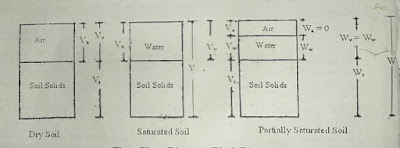Two & Three Phase Diagram For Dry, Moist & Saturated Soil - Civil Engineering

Phase diagram of soil

A soil mass consists of solid particles, containing void space between them. These voids may be filled either by water or by air or both.

Two-Phase Diagram of Soil

The soil will behave as a two-phase system when its void space is filled by either water or air alone. Such condition is possible when the soil is either fully saturated or fully dry.

Three-Phase Diagram of Soil

But, when the soil mass is partially saturated, its void space will be filled up by water as well as air. Under such condition, soil mass will behave a three-phase system. This three-phase system can be easily represented by the block diagram as shown in the figure.Phase Diagram of Soil
The total volume of soil mass (V) consists of the following
V = Vv Vs
V = (V + V) + Vs
Where, Vv = Volume of voids in the soil =Volume of air in the soil (Va)+ Volume of water in the soil (Vw).
V= Volume of solids.